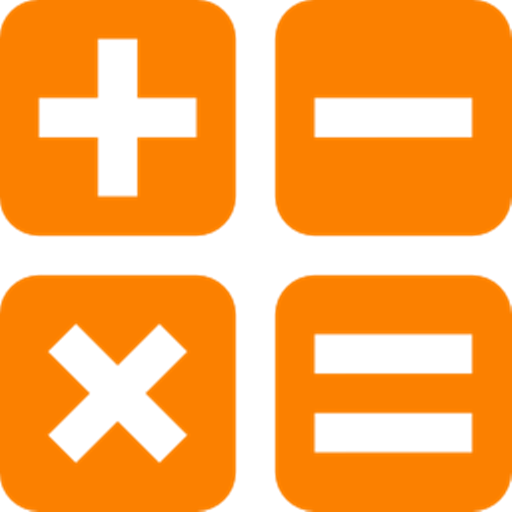## Maths IQ

### by Asvi Mobisol

Free
1666
Categories Tags
###### Description

Maths IQ is a Maths Application designed for checking muliple skills as an individual possesse to have good mathamatics IQ. The app check more than 1000 Maths skill and accordingly give your ranking based on your performance based on time taken and various question rightly answered.

The application is designed in such a way that it is useful for age group from 8 up to adult. With this app you can change the time required

It has tricks to cover entire mathamatics spectrum so that your answer giving skills can be increased. The total tricks counts is more then 250 to name few these are the topics under which we have coverd the trics.

Fun Math Tricks:
2. Subtraction
3. Multiplication table
4. Division
5. Multiply two digit number by 11
6. Square numbers ending in 5
7. Multiply by 5
8. Multiply by 9
9. Multiply by 4
10. Dividing by 5
11. Subtracting from 1000
12. Tough multiplication
13. Power of two
14. Adding numbers close to hundreds
15. Subtracting numbers close to hundreds
16. Multiply numbers between 11 and 19
17. Square numbers between 11 and 99
18. Multiply two digit numbers having same tens digit and ones digits add up to ten
19. Square numbers between 50 and 59
20. Square numbers between 40 and 49
21. Multiply two digit numbers editing in 1
22. Percent
23. Square numbers between 100 and 109

1532
• AOTD

225

1402